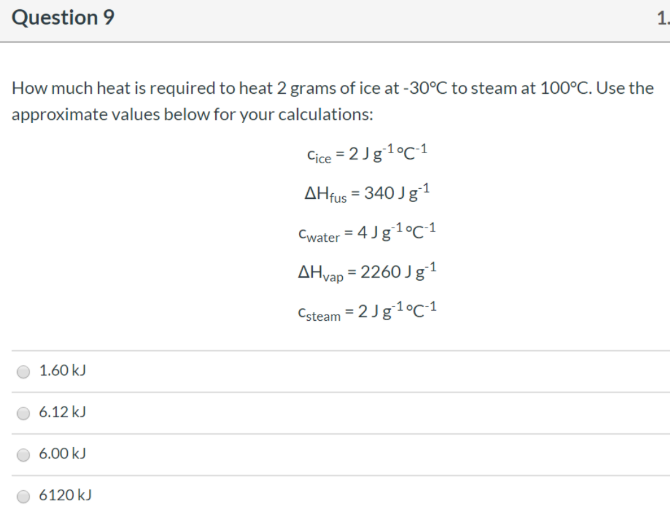# How much heat is required to heat 2 grams of ice at -30°C to steam at 100°C. Use the approximate values below for your calculations: Cice = 2 J g^-1°C^-1 ΔHfus = 340 J g^-1 Cwater = 4 J g^-1 °C^-1 ΔHvap = 2260 J g^-1 Csteam = 2 J g^-1 °C^-1 a) 1.60 kJ b) 6.12 c) 6.00 kJ d) 6120 kJ